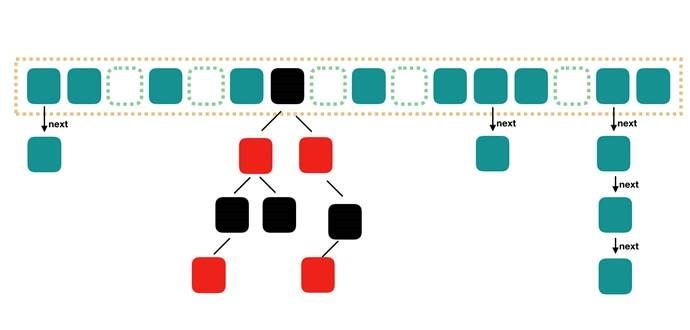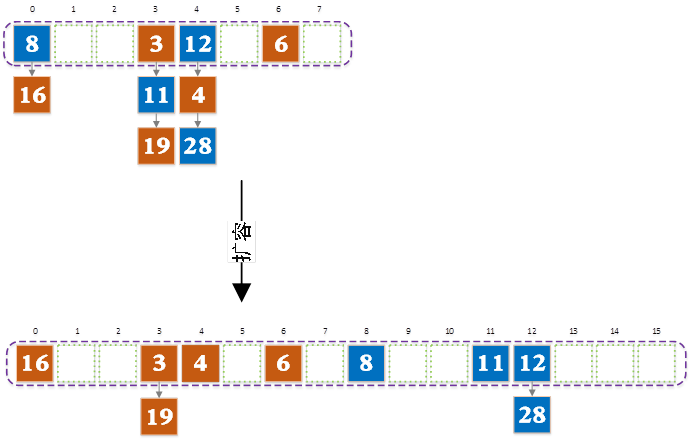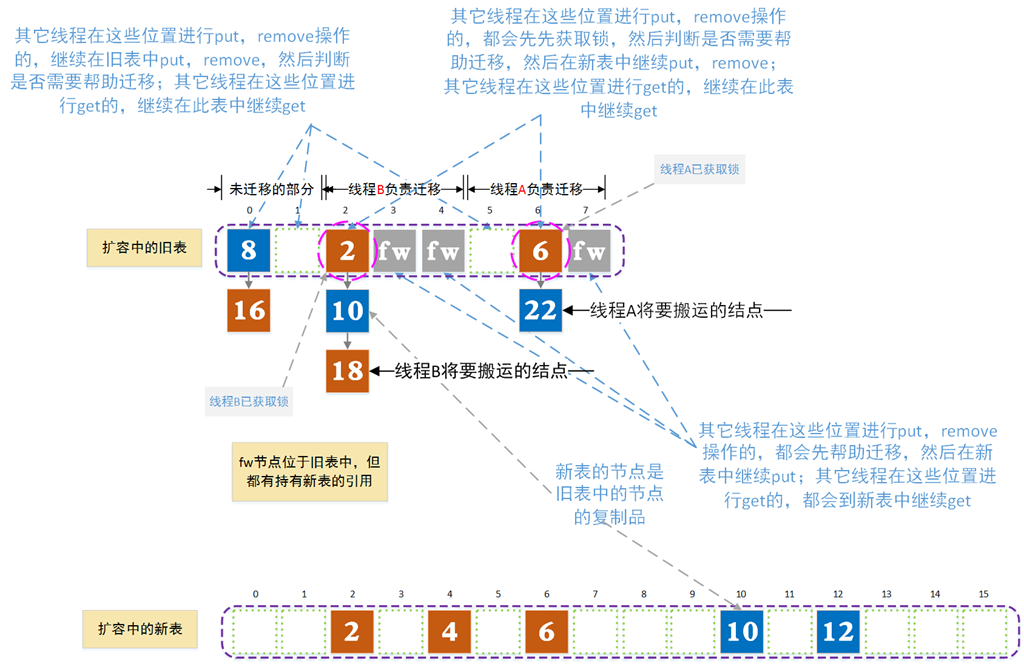# Java8 中 ConcurrentHashMap工作原理的要点分析

#### 1.Java8中 ConcurrentHashMap的结构static class Node<K,V> implements Map.Entry<K,V> {
final int hash;
final K key;
volatile V val;
volatile Node<K,V> next;
//……
//省略其它代码
//……
Node<K,V> find(int h, Object k) {
Node<K,V> e = this;
if (k != null) {
do {
K ek;
if (e.hash == h &&
((ek = e.key) == k || (ek != null && k.equals(ek))))
return e;
} while ((e = e.next) != null);
}
return null;
}
}


#### 2. 表初始化长度与负载因子的含义

public ConcurrentHashMap(int initialCapacity, float loadFactor)

2.1 表的长度

1）在教科书中我们是通过

（Node对象的hash属性值）%表长度

（Node对象的hash属性值）&（表长度-1）

2）在多线程扩容的时，这样的长度设置可以避免在扩容时对新表加锁，从而加快ConcurrentHashMap的扩容速度。关于扩容的细节问题，后面会进行讲述。

2.2 负载因子的含义

#### 3.减少Key的冲突

    static final int HASH_BITS = 0x7fffffff;
static final int spread(int h) {
return (h ^ (h >>> 16)) & HASH_BITS;
}


key.hashCode() &（表长度-1）

#### 4. 扩容操作#### 5. 几个重要方法的源代码分析

5.1 get方法

    public V get(Object key) {
Node<K,V>[] tab; Node<K,V> e, p; int n, eh; K ek;
if ((tab = table) != null && (n = tab.length) > 0 &&
(e = tabAt(tab, (n - 1) & h)) != null) {
if ((eh = e.hash) == h) {
if ((ek = e.key) == key || (ek != null && key.equals(ek)))
return e.val;
}
//说明这个桶迁移已完成 或者 槽中是红黑树的根
else if (eh < 0)
return (p = e.find(h, key)) != null ? p.val : null;
while ((e = e.next) != null) {
if (e.hash == h &&
((ek = e.key) == key || (ek != null && key.equals(ek))))
return e.val;
}
}
return null;
}

5.2 put方法

    public V put(K key, V value) {
return putVal(key, value, false);
}


put方法实际上调用了putVal方法

    final V putVal(K key, V value, boolean onlyIfAbsent) {
if (key == null || value == null) throw new NullPointerException();
int binCount = 0;
for (Node<K,V>[] tab = table;;) {
Node<K,V> f; int n, i, fh;
if (tab == null || (n = tab.length) == 0)
tab = initTable();
//每次循环都会重新计算槽的位置，因为可能刚好完成扩容操作
//扩容完成后会使用新表，槽的位置可能会发生改变
else if ((f = tabAt(tab, i = (n - 1) & hash)) == null) {
//槽为空，先尝试用CAS方式进行添加结点
if (casTabAt(tab, i, null,
new Node<K,V>(hash, key, value, null)))
break;                   // no lock when adding to empty bin
}
//当前线程先帮助迁移,迁移完成后在新表中进行put
else if ((fh = f.hash) == MOVED)
tab = helpTransfer(tab, f);
else {
V oldVal = null;
//加锁操作，防止其它线程对此桶同时进行put,remove,transfer操作
synchronized (f) {
//头结点发生改变，就说明当前链表（或红黑树）的头节点已不是f了
//可能被前面的线程remove掉了或者迁移到新表上了
//如果被remove掉了，需要重新对链表新的头节点加锁
if (tabAt(tab, i) == f) {
//ForwordingNode的hash值为-1
//链表结点的hash值 >= 0
if (fh >= 0) {
binCount = 1;
for (Node<K,V> e = f;; ++binCount) {
K ek;
if (e.hash == hash &&
((ek = e.key) == key ||
(ek != null && key.equals(ek)))) {
oldVal = e.val;
if (!onlyIfAbsent)
e.val = value;
break;
}
Node<K,V> pred = e;
//如果遍历到了最后一个结点，
//那么就说明需要插入新的结点,就把新结点插入在链表尾部
if ((e = e.next) == null) {
pred.next = new Node<K,V>(hash, key,
value, null);
break;
}
}
}
//红黑树的根结点的hash值为-2
else if (f instanceof TreeBin) {
Node<K,V> p;
binCount = 2;
if ((p = ((TreeBin<K,V>)f).putTreeVal(hash, key,
value)) != null) {
oldVal = p.val;
if (!onlyIfAbsent)
p.val = value;
}
}
}
}
if (binCount != 0) {
if (binCount >= TREEIFY_THRESHOLD)
treeifyBin(tab, i);
if (oldVal != null)
return oldVal;
break;
}
}
}
//内部判断是否需要扩容
return null;
}

put方法做了以下几点事情：

1）如果没有初始化就先调用initTable()方法来进行初始化过程2）如果没有hash冲突就尝试CAS方式插入

3）如果还在进行扩容操作就先帮助其它线程进一起行扩容

4）如果存在hash冲突，就加锁来保证put操作的线程安全。

5.3 remove方法

    public V remove(Object key) {
return replaceNode(key, null, null);
}


    final V replaceNode(Object key, V value, Object cv) {
for (Node<K,V>[] tab = table;;) {
Node<K,V> f; int n, i, fh;
if (tab == null || (n = tab.length) == 0 ||
/*每次循环都会重新计算槽的位置，因为在扩容完成后会使用新表
槽的位置可能会发生改变*/
(f = tabAt(tab, i = (n - 1) & hash)) == null)
break;
//如果有线程正在扩容，先帮助它一起扩容，然后在新表中进行put操作
else if ((fh = f.hash) == MOVED)
tab = helpTransfer(tab, f);
else {
V oldVal = null;
boolean validated = false;
//加锁操作，防止其它线程对此桶同时进行put,remove,transfer操作
synchronized (f) {
//头结点发生改变，就说明当前链表（或红黑树）的头节点已不是f了
//可能被前面的线程remove掉了或者迁移到新表上了
//如果被remove掉了，需要重新对链表新的头节点加锁
if (tabAt(tab, i) == f) {
if (fh >= 0) {
validated = true;
for (Node<K,V> e = f, pred = null;;) {
K ek;
if (e.hash == hash &&
((ek = e.key) == key ||
(ek != null && key.equals(ek)))) {
V ev = e.val;
if (cv == null || cv == ev ||
(ev != null && cv.equals(ev))) {
oldVal = ev;
if (value != null)
e.val = value;
else if (pred != null)
pred.next = e.next;
else
setTabAt(tab, i, e.next);
}
break;
}
pred = e;
if ((e = e.next) == null)
break;
}
}
else if (f instanceof TreeBin) {
validated = true;
TreeBin<K,V> t = (TreeBin<K,V>)f;
TreeNode<K,V> r, p;
if ((r = t.root) != null &&
(p = r.findTreeNode(hash, key, null)) != null) {
V pv = p.val;
if (cv == null || cv == pv ||
(pv != null && cv.equals(pv))) {
oldVal = pv;
if (value != null)
p.val = value;
else if (t.removeTreeNode(p))
setTabAt(tab, i, untreeify(t.first));
}
}
}
}
}
if (validated) {
if (oldVal != null) {
if (value == null)
return oldVal;
}
break;
}
}
}
return null;
}


5.4 ForwardingNode

   static final class ForwardingNode<K,V> extends Node<K,V> {
//新表的引用
final Node<K,V>[] nextTable;
ForwardingNode(Node<K,V>[] tab) {
super(MOVED, null, null, null);
this.nextTable = tab;
}

//进行get操作的线程若发现槽中的节点为ForwordingNode类型
//说明该桶中所有结点已迁移完成，会调用ForwordingNode的find方法在新表中进行查找
Node<K,V> find(int h, Object k) {
// loop to avoid arbitrarily deep recursion on forwarding nodes
//从新表中查询
outer: for (Node<K,V>[] tab = nextTable;;) {
//n表示新表的长度
Node<K,V> e; int n;
if (k == null || tab == null || (n = tab.length) == 0 ||
//重新在新表中定位
(e = tabAt(tab, (n - 1) & h)) == null)
return null;
for (;;) {
int eh; K ek;
if ((eh = e.hash) == h &&
((ek = e.key) == k || (ek != null && k.equals(ek))))
return e;
//继续递归查询？这里没看懂
if (eh < 0) {
if (e instanceof ForwardingNode) {
tab = ((ForwardingNode<K,V>)e).nextTable;
continue outer;
}
else
return e.find(h, k);
}
//下一个
if ((e = e.next) == null)
return null;
}
}
}
}


ForwardingNode类继承了Node类，所以ForwardingNode对象也是Node类型对象，所以它也可以放到表中。

ForwardingNode在扩容中使用。每一个ForwardingNode对象都包含扩容后的表的引用（新表保存在nextTable属性中）。 ForwardingNode对象的key，value，next属性值全部为null，它的hash值为-1（注意小于0哦，可以去看看get方法中对应的部分了）。

ForwardingNode对象中定义了find的方法,它是从扩容后的新表中查询结点，而不是以自身为头结点进行查找。

5.5 扩容方法

    private final void transfer(Node<K,V>[] tab, Node<K,V>[] nextTab) {
int n = tab.length, stride;
if ((stride = (NCPU > 1) ? (n >>> 3) / NCPU : n) < MIN_TRANSFER_STRIDE)
stride = MIN_TRANSFER_STRIDE; // subdivide range
if (nextTab == null) {            // initiating
try {
@SuppressWarnings("unchecked")
Node<K,V>[] nt = (Node<K,V>[])new Node<?,?>[n << 1];
nextTab = nt;
} catch (Throwable ex) {      // try to cope with OOME
sizeCtl = Integer.MAX_VALUE;
return;
}
nextTable = nextTab;
transferIndex = n;
}
int nextn = nextTab.length;
ForwardingNode<K,V> fwd = new ForwardingNode<K,V>(nextTab);
boolean finishing = false; // to ensure sweep before committing nextTab
for (int i = 0, bound = 0;;) {
Node<K,V> f; int fh;
int nextIndex, nextBound;
if (--i >= bound || finishing)
else if ((nextIndex = transferIndex) <= 0) {
i = -1;
}
else if (U.compareAndSwapInt
(this, TRANSFERINDEX, nextIndex,
nextBound = (nextIndex > stride ?
nextIndex - stride : 0))) {
bound = nextBound;
i = nextIndex - 1;
}
}
if (i < 0 || i >= n || i + n >= nextn) {
int sc;
if (finishing) {
nextTable = null;
table = nextTab;
sizeCtl = (n << 1) - (n >>> 1);
return;
}
if (U.compareAndSwapInt(this, SIZECTL, sc = sizeCtl, sc - 1)) {
if ((sc - 2) != resizeStamp(n) << RESIZE_STAMP_SHIFT)
return;
i = n; // recheck before commit
}
}
else if ((f = tabAt(tab, i)) == null)
advance = casTabAt(tab, i, null, fwd);
else if ((fh = f.hash) == MOVED)
else {
//头结点加锁，防止其它线程此时对该桶进行put和remove操作
synchronized (f) {
//和put及remove操作判断头结点是否改变的原理类似
if (tabAt(tab, i) == f) {
// fh >= 0 表示链表
Node<K,V> ln, hn;
if (fh >= 0) {
int runBit = fh & n;
Node<K,V> lastRun = f;
for (Node<K,V> p = f.next; p != null; p = p.next) {
int b = p.hash & n;
if (b != runBit) {
runBit = b;
lastRun = p;
}
}
if (runBit == 0) {
ln = lastRun;
hn = null;
}
else {
hn = lastRun;
ln = null;
}
//按新表中槽的位置分为两部分
//注意新表中的节点都是新建的，而不是修改原的结点的next指针
//这样做是为了同其它线程的get方法并发时能get正确的结果
for (Node<K,V> p = f; p != lastRun; p = p.next) {
int ph = p.hash; K pk = p.key; V pv = p.val;
if ((ph & n) == 0)
ln = new Node<K,V>(ph, pk, pv, ln);
else
hn = new Node<K,V>(ph, pk, pv, hn);
}
setTabAt(nextTab, i, ln);
setTabAt(nextTab, i + n, hn);
//将头结点设置为fwd
setTabAt(tab, i, fwd);
}
//表示红黑树
else if (f instanceof TreeBin) {
TreeBin<K,V> t = (TreeBin<K,V>)f;
TreeNode<K,V> lo = null, loTail = null;
TreeNode<K,V> hi = null, hiTail = null;
int lc = 0, hc = 0;
for (Node<K,V> e = t.first; e != null; e = e.next) {
int h = e.hash;
TreeNode<K,V> p = new TreeNode<K,V>
(h, e.key, e.val, null, null);
if ((h & n) == 0) {
if ((p.prev = loTail) == null)
lo = p;
else
loTail.next = p;
loTail = p;
++lc;
}
else {
if ((p.prev = hiTail) == null)
hi = p;
else
hiTail.next = p;
hiTail = p;
++hc;
}
}
ln = (lc <= UNTREEIFY_THRESHOLD) ? untreeify(lo) :
(hc != 0) ? new TreeBin<K,V>(lo) : t;
hn = (hc <= UNTREEIFY_THRESHOLD) ? untreeify(hi) :
(lc != 0) ? new TreeBin<K,V>(hi) : t;
setTabAt(nextTab, i, ln);
setTabAt(nextTab, i + n, hn);
//将头结点设置为fwd
setTabAt(tab, i, fwd);
}
}
}
}
}
}


1）构建一个nextTable,它的容量是原来的两倍，这个操作是单线程完成的。

2）是将原来table中的结点迁移到nextTable中，这里允许多线程进行操作。

private transient volatile int transferIndex;

transfer函数中定义一个局部变量stride，它表示了每个线程的一次迁移处理的桶的个数，当一个线程处理完成后 transferIndex就自减一个stride，那么下一个线程就应该从transerIndex – stride处开始，往前处理stride个桶，以此类推完成协作。为什么要设计成从旧表的后部开始往头部的方向搬运呢？个人猜想是搬运结束的时候条件是统一的，只是写代码的技巧吧。当然怎么确定整个旧表上的内容全部都迁移了，还需要读更多的源代码，这里就不作分析了。

#### 6.并发问题的分析6.1初化的同步问题

private transient volatile int sizeCtl;

U.compareAndSwapInt(this, SIZECTL, sc, -1)

6.2 put方法和remove方法之间的同步问题

6.3 put(或remove)方法和get方法的同步问题

6.4 get方法和扩容操作的同步问题

1）该位置的头结点是Node类型对象，直接get，即使这个桶正在进行迁移，在get方法未完成前，迁移操作已完成，即槽被设置成了ForwordingNode对象，也没关系，并不影响get的结果。因为get线程仍然持有旧链表的引用，可以从当前结点位置访问到所有的后续结点。这是因为新表中的节点是通过复制旧表中的结点得到的，所以新表的结点的next值不会影响旧表中对应结点的next值。当get方法结束后，旧链表就出于不可达的状态，会被垃圾回收线程回收。

2）该位置的头结点是ForwordingNode类型对象（头结点的hash值 == -1），头结点是ForwordingNode类型的对象，调用该对象的find方法，在新表中查找。

6.5 put(或remove)方法和扩容操作的同步问题